# Practice Questions with Solutions: Addition and Subtraction Notes | Study Mathematics for Class 4 - Class 4

## Class 4: Practice Questions with Solutions: Addition and Subtraction Notes | Study Mathematics for Class 4 - Class 4

The document Practice Questions with Solutions: Addition and Subtraction Notes | Study Mathematics for Class 4 - Class 4 is a part of the Class 4 Course Mathematics for Class 4.
All you need of Class 4 at this link: Class 4

Question 1: Add 4,032 and 1, 74 6.
Solution :

Step 1. Arrange the numbers in columns.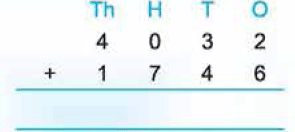Step 2. Adding ones, we have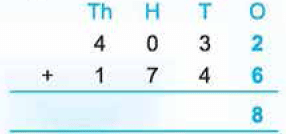Step 3. Adding tens, we have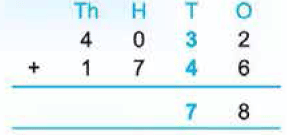Step 4. Adding hundreds, we have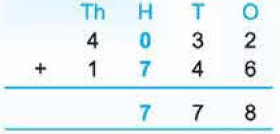Step 5. Adding thousands, we have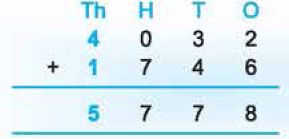Hence, 4,032 + 1, 74 6 = 5, 778.
(Five thousand, seven hundred seventy-eight).

Question 2: Add 5,386 and 3,927.
Solution:
Step 1. Arrange the numbers in columns.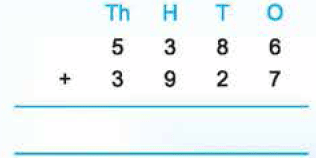Step 2. Adding ones, we have 6 ones + 7 ones = 13 ones = 1 ten + 3 ones. Carry over 1 to tens column and write 3 under ones column.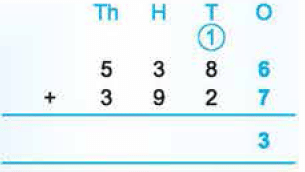Step 3. Adding tens, we have
1 ten (carried) + 8 tens + 2 tens = 11 tens = 1 hundred + 1 ten.
Carry over 1 to hundreds column and write 1 under tens column.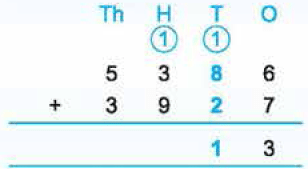Step 4. Adding hundreds, we have 1 hundred (carried) + 3 hundreds + 9 hundreds = 13 hundreds = 1 thousand + 3 hundreds. Carry over 1 to thousands column and write 3 under hundreds column.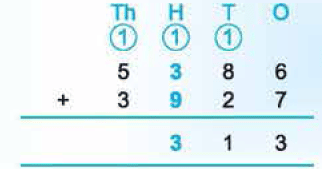Step 5. Adding thousands, we have 1 thousand (carried) + 5 thousands + 3 thousands = 9 thousands.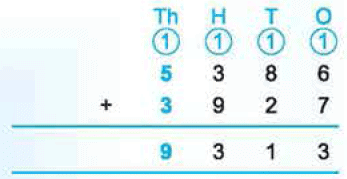Hence,
5,386 + 3,927 = 9,313
(Nine thousand, three hundred thirteen).

Question 3: The population of town A is 2,301, the population of town B is 4,875, the population of town C is 1, 835. What is the total population of the three towns?
Solution :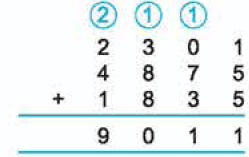Population of town A = 2,301
Population of town B = 4,875
Population of town C = 1,835
Total population of the three towns
= 2,301 + 4,875 + 1, 835 = 9,011.
Hence, the total population of the three towns = 9,011.

Question 4: Add 7,521; 1 ,025 and 1 ,352.
Solution :

Step 1. Arrange the numbers in columns.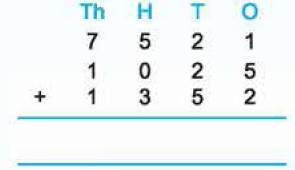Step 2. Adding ones, we have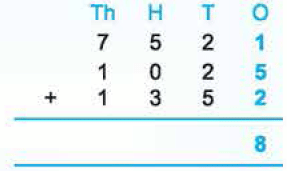Step 3. Adding tens, we have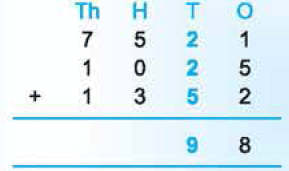Step 4. Adding hundreds, we have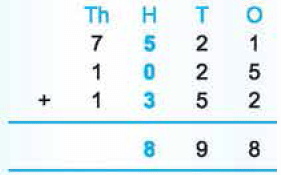Step 5. Adding thousands, we have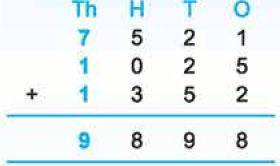Hence, 7,521 + 1,025 + 1,352 = 9,898
(nine thousand, eight hundred ninety-eight).

Question 5: Add 2,908, 6,123 and 254
Solution :

Step 1.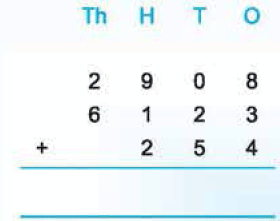Step 2.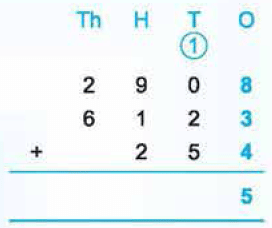Step 3.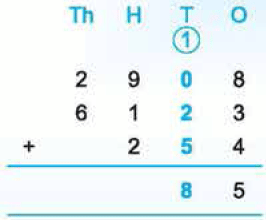Step 4.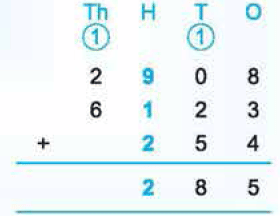Step 5.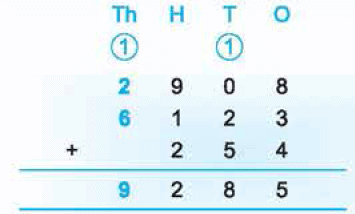Hence,
2,908 + 6,123 + 254 = 9,285

(Nine thousand, two hundred eighty-five).

Question 6: Which number is 528 more than 7,874?
Solution :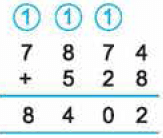The required number = 7,874 + 528 = 8,402. Hence, the required number is 8,402.

Question 7: Simplify : 7,530 + 1, 817 - 5,173
Solution :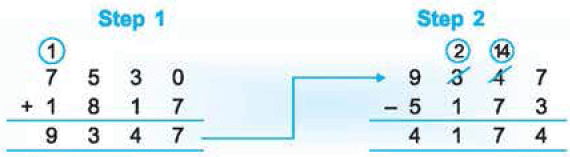Hence, 7,530 + 1,817- 5,173 = 4,174.

Question 8: 3,856 - 2,385 + 6,389 - 987
Solution :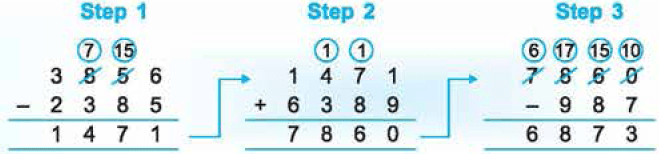Hence, 3,856 - 2,385 + 6,389 - 987 = 6,873

Question 9: Subtract 5,013 from 8,347.
Solution :

Step 1. Arrange the numbers in columns.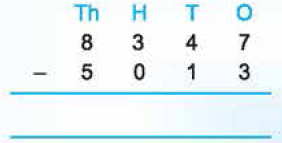Step 2. Subtracting ones, we have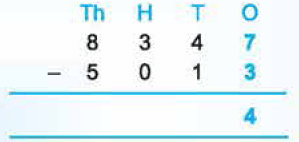Step 3. Subtracting tens, we have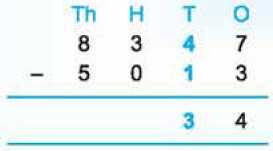Step 4. Subtracting hundreds, we have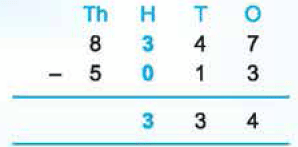Step 5. Subtracting thousands, we have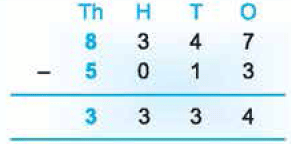Hence, 8,347 - 5,013 = 3,334
(Three thousand, three hundred thirty-four).

Question 10: A factory produced 3,263 mercury lamps in 2012. In 2013, it produced 4,450 mercury lamps. By how much did the factory's production increase?
Solution :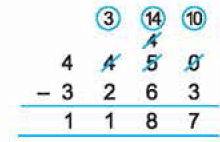Production in 2013 = 4,450
Production in 2012 = 3,263
Increase in production = 4,450 - 3,263
= 11187.
Hence, the production increased by 1,187.

Question 11: Subtract 2,856 from 8, 153.
Solution :

Step 1. Arrange the numbers in columns.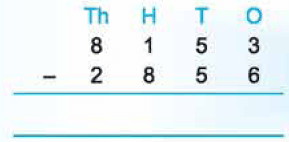Step 2. Subtract the ones. Here 3 < 6, so regroup 5 tens 3 ones as 4 tens and 13 ones.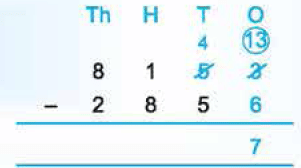Step 3. Subtract the tens. Here, 4 < 5, so, regroup 1 hundred 4 tens as 0 hundreds and 14 tens.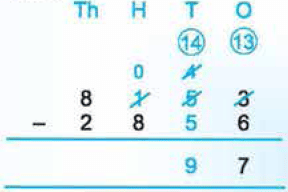Step 4. Subtract the hundreds. Here, 0 < 8. so regroup 8 thousands 0 hundreds as 7 thousand and 10  hundreds.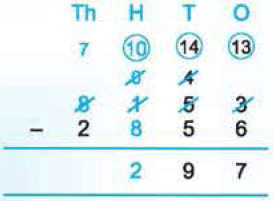Step 5. Subtract the thousands.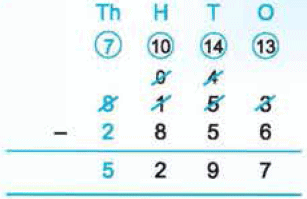Hence, 8,153 - 2,856 = 5,297
(Five thousand, two hundred ninety-seven).

Question 12: What must be added to 4,213 to get 7,631?
Solution :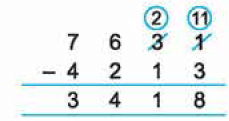The sum of two numbers = 7,631.
One number= 4,213
The other number= 7,631 - 4,213
= 3,418
Hence, the required number = 3,418.

Question 13: Subtract: 9,000 - 6.124.
Solution:

Step 1. Arrange the numbers in columns.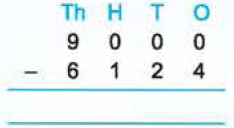Step 2. Regroup 9 thousands.
9 thousands = 8 thousands + 10 hundreds
10 hundreds = 9 hundreds + 10 tens
10 tens = 9 tens + 10 ones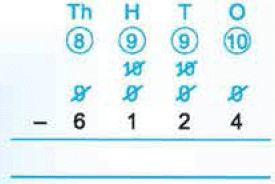Step 3. Subtract the ones.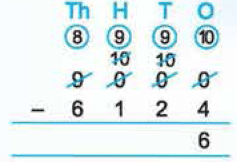Step 4. Subtract the tens.Step 5. Subtract the hundreds.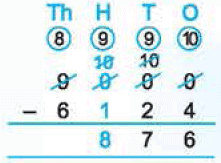Step 6. Subtract the thousands.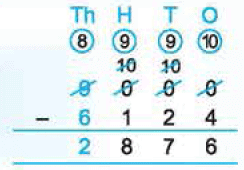Hence, 9,000 - 6,124 = 2,876.

The document Practice Questions with Solutions: Addition and Subtraction Notes | Study Mathematics for Class 4 - Class 4 is a part of the Class 4 Course Mathematics for Class 4.
All you need of Class 4 at this link: Class 4Use Code STAYHOME200 and get INR 200 additional OFF

## Mathematics for Class 4

15 videos|68 docs|32 tests

Track your progress, build streaks, highlight & save important lessons and more!

,

,

,

,

,

,

,

,

,

,

,

,

,

,

,

,

,

,

,

,

,

;